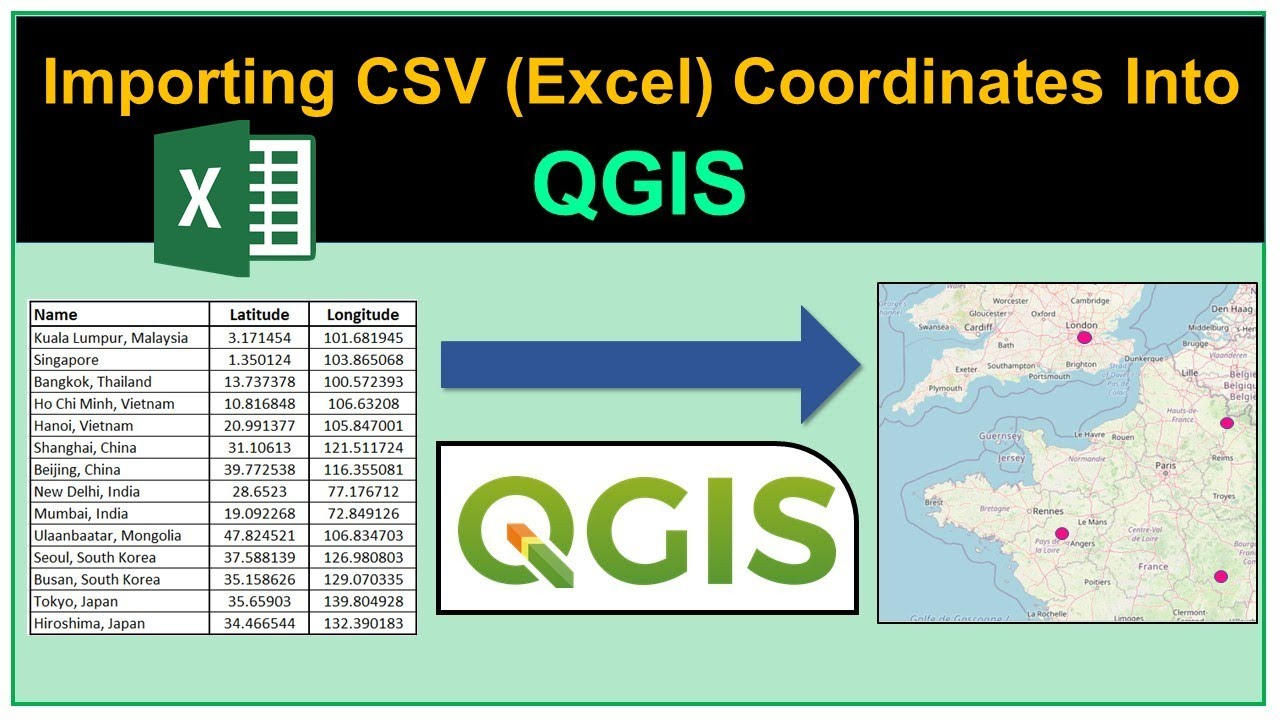# Importing CSV (Excel) Coordinates into QGISIn this tutorial, we will show you how to import comma separated value (CSV) data which contains latitude & longitude into QGIS, how to plot the points on a map, and how to export the data into an ESRI Shapefile.

In this tutorial, we will show you how to import comma separated value (CSV) data which contains latitude & longitude into QGIS, how to plot the points on a map, and how to export the data into an ESRI Shapefile.

## Data Validation in Excel

What is Data Validation in Excel. Data validation is a feature in Excel used to control what a user can enter into a cell. Learn more about it with Examples.

Advanced Excel Online Training & Certification Courses by CETPA with Real time projects. Enroll now to get student offer of 50% on Online Course. Call us: 9212172602.

## Microsoft Excel Tutorial - Objects In Ms Excel- Excel Worksheet Objects

In this MS Excel tutorials, video we are going to see the differnce between tables and cell ranges in MS Excel. Also we are going to see that how can we create a Table from data given to us and what all changes we can apply to our tables.

## Microsoft Excel Tutorial - Slicers on Charts in MS Excel.

In this MS Excel tutorials, video we are going to apply the slicers in charts in MS Excel. However, there is no direct method of doing so, that is why we are going to use a simple trick over here. Also we are going to see that how can we connect same slicer with multiple charts in MS Excel. ​

## Excel VBA Tutorial for Beginners 19 - VBA Insert Cells in MS Excel

In this MS Excel VBA video, we are going to see the usage of the Insert Cells feature of MS Excel. Using this feature we can insert cells at some specified locations easily. In this video we will see the Overview of formulas in Excel.